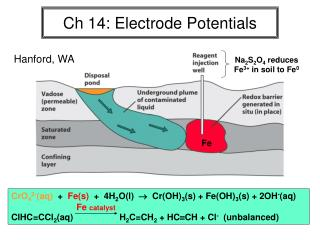DownloadDownload PresentationCh 14: Electrode Potentials

# Ch 14: Electrode Potentials

Télécharger la présentation## Ch 14: Electrode Potentials

- - - - - - - - - - - - - - - - - - - - - - - - - - - E N D - - - - - - - - - - - - - - - - - - - - - - - - - - -
##### Presentation Transcript

1. Ch 14: Electrode Potentials Hanford, WA Na2S2O4 reduces Fe3+ in soil to Fe0 Fe CrO42-(aq) + Fe(s) + 4H2O(l)  Cr(OH)3(s) + Fe(OH)3(s) + 2OH-(aq)ClHC=CCl2(aq) H2C=CH2 + HCCH + Cl- (unbalanced) Fe catalyst

2. Redox Reaction Review 8H+ + Cr2O72- + 3H2S  2Cr3+ + 7H2O + 3S ox ½ rxn: red ½ rxn: reducing agent = oxidizing agent =

3. Some Terminology Current in Amp = C/s Volt (emf) = 1 Joule/Coulomb (J/C) Charge on electron (e) = 1.60 x 10-19 C Faraday (F) = charge on a mole of e's = NA x e = 96,485 C/mol

4. Galvanic Cells (Sec 14-2) • Be able to: • draw the line diagram and cell • identify cathode and anode and 1/2 rxn's • determine electrode polarities & direction of electron flow • give the net cell reaction • Calculate Eocell, E, & K Zn | ZnCl2(1.0M) || CuSO4(1.0M) | Cu(s)

5. Example p. 315: Write a line diagram for the given cell. Write the half reactions and the net cell reaction.

6. Standard Potentials (Sec 14-3) Standard Hydrogen Electrode (SHE): aH+ = 1.00 M PH2 = 1.00 atm Eo = 0.00 V (by definition)

7. a more complete table -

8. Back to the Zn/Cu galvanic cell: Zn | ZnCl2(1.0M) || CuSO4(1.0M) | Cu(s) E > 0 spontaneous E = 0 equilibrium E < 0 spontaneous reverse direction

9. The Nernst Equation (Sec 14-4)calculating the non-standard cell potential for aA + bB = cC + dD, from Thermodynamics we know - G

10. Example p. 321: Find the voltage of the cell in Figure 14-4 if the right half-cell contains 0.50M KCl(aq) and the left half-cell contains 0.010M Cd(NO3)2(aq). Write the net cell reaction and state whether it is spontaneous in the forward or reverse direction.

11. K Eo and the Equilibrium Constant (Sec 14-5) aA + bB = cC + dD at equilibrium -

12. Example p. 326: Find the equilibrium constant for the reactionFe3+ + Ag(s) + Cl- = Fe2+ + AgCl(s), which is the net cell reaction in Fig. 14-8.

13. Electrochemical Measurement of Kw

14. Reference Electrodes (Sec. 14-6) The Standard Hydrogen Electrode (SHE) is inconvenient to use, so other more practical electrodes are commonly used in the lab and in the field. Their half-potentials have been measured relative to the SHE. Ag/AgCl Reference Electrode AgCl(s) + e = Ag(s) + Cl- Eo = +0.222 V (1.0 M KCl)= +0.197 V (sat’d KCl)

15. Calomel Reference Electrode ½Hg2Cl2(s) + e = Hg(l) + Cl- Eo = +0.268 V (1.0 M KCl) = +0.241 V (sat’d KCl)

16. Voltage Conversions Between Different Reference Scales